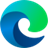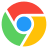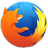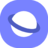# scaleX() CSS Function

### Description

The CSS `scaleX()` function is used to scale an element along the x-axis (horizontally). It takes a single parameter, which is a number that represents the scaling factor. A scaling factor of 1 means that the element will not be scaled at all, while a scaling factor of 2 means that the element will be doubled in width. A scaling factor of less than 1 will reduce the width of the element.

The `scaleX()` function can be used to create a variety of effects, such as zooming in or out on an element, or creating a distorted or warped effect. It can also be used to create animations, such as making an element grow or shrink over time.

Here is an example of how to use the `scaleX()` function:

``````/* Scale an element to twice its width */
.my-element {
transform: scaleX(2);
}
``````

Here is another example of how to use the `scaleX()` function to create an animation:

``````/* Scale an element from 0 to 1 over 2 seconds */
.my-element {
transform: scaleX(0);
transition: transform 2s;
}

.my-element:hover {
transform: scaleX(1);
}
``````

This code will cause the element to be initially scaled to 0 width. When the user hovers over the element, it will gradually scale over 2 seconds to its original size.

### Syntax

`scaleX() = transform: scaleX(<number>);`

### Values

• <number>The value is a positive or negative number.
- a value greater than 1 (for example: 1.5) increases the scale;
- a value less than 1 (for example: 0.8) reduces the scale;
- a negative value (for example: -1) mirrors the element horizontally;
- a value of 1 leaves the element size unchanged and does not give a visible effect.

### Example

``<img class="scaleX" src="images/nature.jpg" alt="Nature Image">``
``````.scaleX {
transform: scaleX(2);
}``````

### Browser Support

The following table will show you the current browser support for the CSS `scaleX()` function.

 Desktop12 1 3.5 10.1 3.5
 Tablets / Mobile18 4 11 3.2 1 2

Last updated by CSSPortal on: 7th October 2023